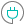#FDIST (PQL - xl)

This function calculates the F probability distribution, to see degrees of diversity between two sets of data

#### Syntax

FDIST(x, deg freedom1, deg freedom2)

##### Function Arguments
 Name Description Type Optional x Value at which to evaluate the function Number deg freedom1 Number of degrees of freedom for the numerator; if not an integer, the number is truncated Number deg freedom2 Number of degrees of freedom for the denominator; if not an integer, the number is truncated Number